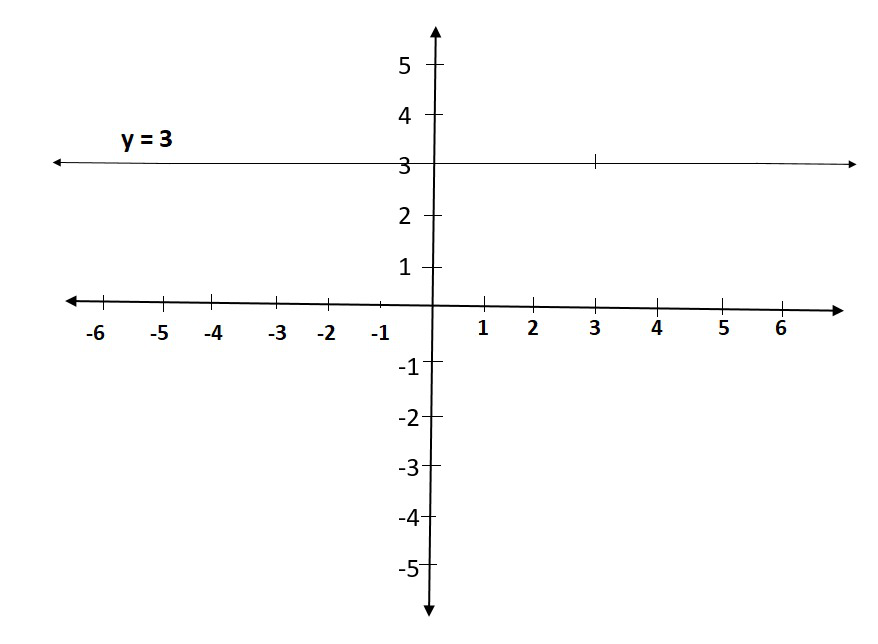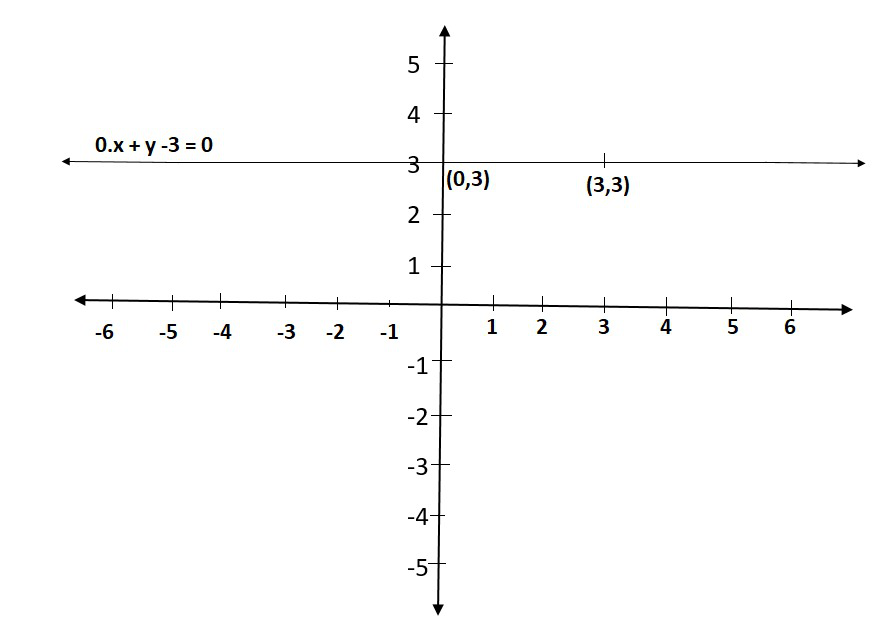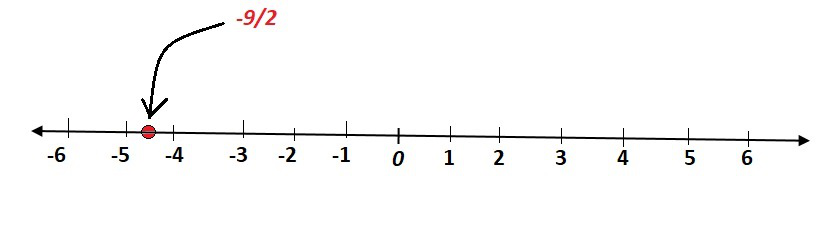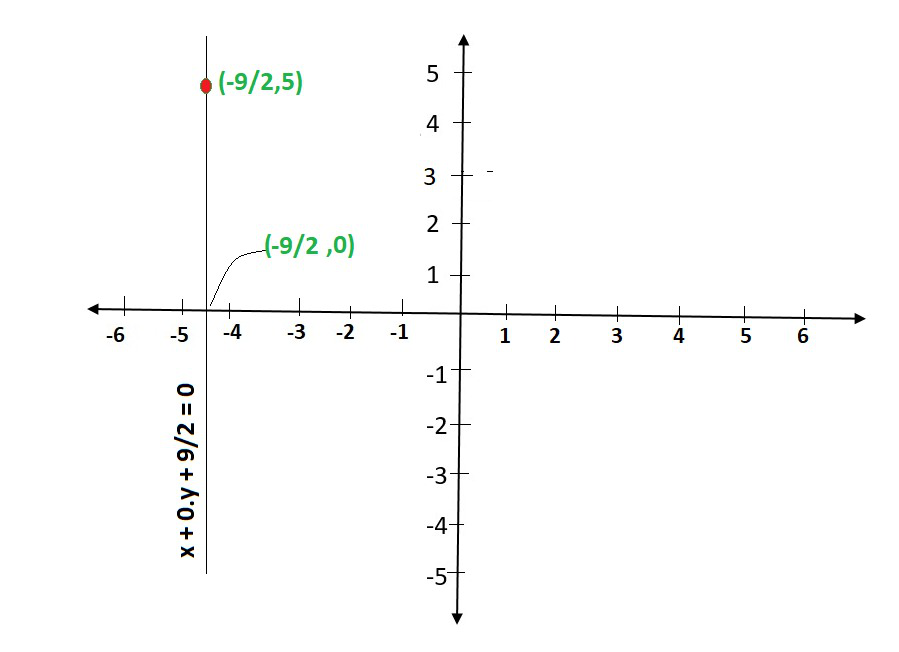Open In App
Related Articles
• NCERT Solutions for Class 9 Maths

# Class 9 NCERT Solutions- Chapter 4 Linear Equations in two variables – Exercise 4.4

### Question 1. Give the geometric representations of y = 3 as an equation

(i) in one variable

(ii) in two variables

Solution:

(i) If y = 3 is treated as a equation in one variable y only, then it has the unique solution

y = 3, which is a point on the number line(ii) When an equation in two variables, it can be expressed as,

0.x + y – 3 = 0

which is a linear equation in the variables x and y. This is represented by a line. Now all the values of x are permissible because 0.x is always 0. However, y must satisfy the equation y = 3

This has infinitely many solutions. In fact, they are all of the form (r, 3), where r is any real number, so

We can have these two solutions for making line on a graph as:### Question 2. Give the geometric representations of 2x + 9 = 0 as an equation

(i) in one variable

(ii) in two variables

Solution:

Firstly, we solve 2x + 9 = 0, to get

x = -9/2

(i) If x = -9/2 is treated as a equation in one variable x only, then it has the unique solution

x = -9/2, which is a point on the number line.(ii) When an equation in two variables, it can be expressed as,

x + 0.y + 9/2 = 0

which is a linear equation in the variables x and y. This is represented by a line. Now all the values of y are permissible because 0.y is always 0. However, x must satisfy the equation x = -9/2

This has infinitely many solutions. In fact, they are all of the form (-9/2, r), where r is any real number, so

We can have these two solutions for making line on a graph as: